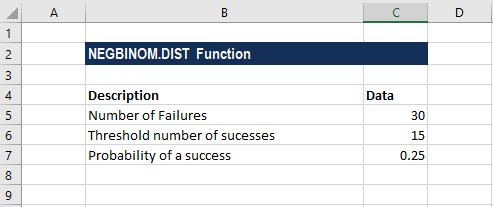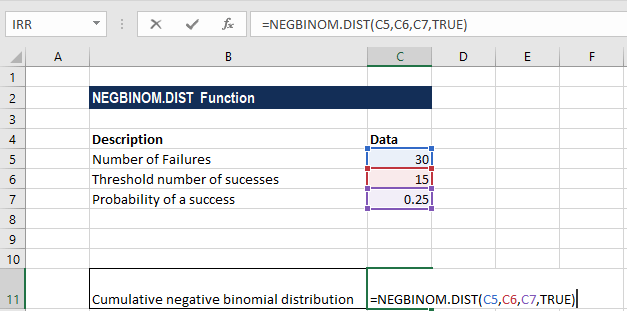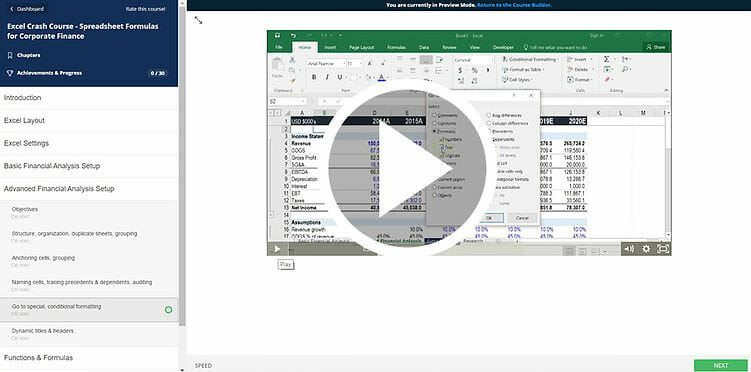# NEGBINOM.DIST Function

Calculates the negative binomial distribution probability

## Negative Binomial Distribution Formula

The NEGBINOM.DIST Function is categorized under Excel Statistical functions. It will calculate the negative binomial distribution probability. It determines the probability mass function or the cumulative distribution function for a negative binomial distribution. For example, using the function, we can find out the probability that when a coin is tossed, we will get 10 heads before we get 12 tails. This guide will break down the Excel negative binomial distribution formula.

For the negative binomial distribution, we will fix the number of successes but use a variable number of trials. NEGBINOM.DIST will calculate the probability of a given number of failures occurring before a fixed number of successes.

The two types of the function that are used are as follows:

1. The Probability Mass Function – This calculates the probability of there being exactly “f” failures before “s” successes.
2. The Cumulative Distribution Function – This calculates the probability of there being at most “f” failures before “s” successes.

In financial analysis, the NEGBINOM.DIST function can be useful for finding out the probability of getting x return from a stock or portfolio before getting a negative return.

### Negative Binomial Distribution Formula

=NEGBINOM.DIST(number_f,number_s,probability_s,cumulative)

The NEGBINOM.DIST function uses the following arguments:

1. Number_f (required argument) – This is the number of failures that are encountered before number_s successes.
2. Number_s (required argument) – The required number of successes.
3. Probability_s (required argument) – This is the probability of successes in one trial.
4. Cumulative (optional argument) – This specifies the type of negative binomial distribution formula to be calculated. It can either be TRUE (uses the cumulative distribution function) or FALSE (use the probability mass function).

### How to use the Negative Binomial Distribution Formula in Excel?

To understand the uses of the NEGBINOM.DIST function, let us consider an example:

#### Example – Negative Binomial Distribution Formula

Suppose we are given the following data:The formula for calculating the binomial distribution is shown below:We get the result below:

### Notes about the Negative Binomial Distribution in Excel

1. The NEGBINOM.DIST function will truncate all numerical values to integers.
2. #VALUE! error – Occurs when:
• The number_f, number_s, or probability_s arguments are not recognized as numeric values;
• The cumulative argument provided is not recognized as a numeric or a logical value.
3. #NUM! error – Occurs when:
• The number_f provided is less than 0 or the supplied number_s is greater than 1.
• The probability_s provided is less than 0 or greater than 1.
4. The NEGBINOM.DIST function was introduced in MS Excel 2010 and hence is unavailable in earlier versions. For older versions, we can use the NEGBINOMDIST function.

### Free Excel Course

Check out CFI’s Free Excel Course if you want to learn more about Excel functions using your own online instructor. You’ll receive step by step instructions and demonstrations on how to become an expert in Excel.Thanks for reading CFI’s guide to important Excel functions! By taking the time to learn and master these functions, you’ll significantly speed up your financial analysis. To learn more, check out these additional CFI resources:

• Excel Functions for Finance